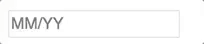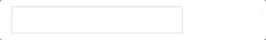## react-number-format

React component to format number in an input or as a text.

### Features

1. Prefix, suffix and thousand separator.
2. Custom format pattern.
4. Custom formatting handler.
5. Formatting a input or a simple text.

### InstallThrough npm
`npm install react-number-format --save`

Or get compiled development and production version from ./dist

### Usage

ES6

``````import NumberFormat from 'react-number-format';
``````

ES5

``````const NumberFormat = require('react-number-format');
``````

Typescript

``````import * as NumberFormat from 'react-number-format';
``````

### Props

Props Options Default Description
thousandSeparator mixed: single character string or boolean true (true is default to ,) none Add thousand separators on number
decimalSeparator single character string . Support decimal point on a number
decimalScale number none If defined it limits to given decimal scale
fixedDecimalScale boolean false If true it add 0s to match given decimalScale
allowNegative boolean true allow negative numbers (Only when format option is not provided)
allowEmptyFormatting boolean true Apply formatting to empty inputs
prefix String (ex : \$) none Add a prefix before the number
suffix String (ex : /-) none Add a suffix after the number
value Number or String null Value to the number format. It can be a float number, or formatted string. If value is string representation of number (unformatted), isNumericString props should be passed as true.
isNumericString boolean false If value is passed as string representation of numbers (unformatted) then this should be passed as true
displayType String: text / input input If input it renders a input element where formatting happens as you input characters. If text it renders it as a normal text in a span formatting the given value
type One of ['text', 'tel'] text Input type attribute
format String : Hash based ex (#### #### #### ####)
Or Function
none If format given as hash string allow number input inplace of hash. If format given as function, component calls the function with unformatted number and expects formatted number.
removeFormatting (formattedValue) => numericString none If you are providing custom format method and it add numbers as format you will need to add custom removeFormatting logic
mask String (ex : _) `' '` If mask defined, component will show non entered placed with masked value.
customInput Component Reference input This allow supporting custom inputs with number format.
onValueChange (values, e) => {} none onValueChange handler accepts values object and event object (change / blur) as second parameter
isAllowed (values) => true or false none A checker function to check if input value is valid or not
renderText (formattedValue) => React Element null A renderText method useful if you want to render formattedValue in different element other than span.
getInputRef (elm) => void null Method to get reference of input or span based on displayType prop. See Getting reference

Other than this it accepts all the props which can be given to a input or span based on displayType you selected.

#### values object

values object is on following format

``````{
formattedValue: '\$23,234,235.56', //value after applying formatting
value: '23234235.56', //non formatted value as numeric string 23234235.56, if your are setting this value to state make sure to pass isNumericString prop to true
floatValue: 23234235.56 //floating point representation. For big numbers it can have exponential syntax
}
``````

Its recommended to use formattedValue / value / floatValue based on the initial state (it should be same as the initial state format) which you are passing as value prop. If you are saving the `value` key on state make sure to pass isNumericString prop to true.

### Notes and quirks

1. Value can be passed as string or number, but if it is passed as string it should be either formatted value or if it is a numeric string, you have to set isNumericString props to true.

2. Value as prop will be rounded to given decimal scale if format option is not provided.

3. If you want to block floating number set decimalScale to 0.

4. Use type as tel when you are providing format prop. This will change the mobile keyboard layout to have only numbers. In other case use type as text, so user can type decimal separator.

5. onChange no longer gets values object. You need to use onValueChange instead. onChange/onFocus/onBlur and other input events will be directly passed to the input.

6. Its recommended to use formattedValue / value / floatValue based on the initial state (it should be same as the initial state format) which you are passing as value prop. If you are saving the `value` key on state make sure to pass isNumericString prop to true.

7. onValueChange is not same as onChange. It gets called on different events. So don't make assumption about the event object (second parameter). It can be change event or blur event.

### Examples

#### Prefix and thousand separator : Format currency as text

``````var NumberFormat = require('react-number-format');

<NumberFormat value={2456981} displayType={'text'} thousandSeparator={true} prefix={'\$'} />
``````

Output : \$2,456,981

#### Custom renderText method

``````var NumberFormat = require('react-number-format');

<NumberFormat value={2456981} displayType={'text'} thousandSeparator={true} prefix={'\$'} renderText={value => <div>{value}</div>} />
``````

Output : `<div> \$2,456,981 </div>`

#### Format with pattern : Format credit card as text

``````<NumberFormat value={4111111111111111} displayType={'text'} format="#### #### #### ####" />
``````

Output : 4111 1111 1111 1111

#### Prefix and thousand separator : Format currency in input

``````<NumberFormat thousandSeparator={true} prefix={'\$'} />
``````#### Maintaining change value on state

``````<NumberFormat value={this.state.profit} thousandSeparator={true} prefix={'\$'} onValueChange={(values) => {
const {formattedValue, value} = values;
// formattedValue = \$2,223
// value ie, 2223
this.setState({profit: formattedValue})
}}/>
``````

#### Format with pattern : Format credit card in an input

``````<NumberFormat format="#### #### #### ####" />
``````#### Format with mask : Format credit card in an input

``````<NumberFormat format="#### #### #### ####" mask="_"/>
``````#### Format with mask as array

Mask can also be a array of string. Each item corresponds to the same index #.

``````<NumberFormat format="##/##" placeholder="MM/YY" mask={['M', 'M', 'Y', 'Y']}/>
``````#### Custom format method : Format credit card expiry time

``````function limit(val, max) {
if (val.length === 1 && val > max) {
val = '0' + val;
}

if (val.length === 2) {
if (Number(val) === 0) {
val = '01';

//this can happen when user paste number
} else if (val > max) {
val = max;
}
}

return val;
}

function cardExpiry(val) {
let month = limit(val.substring(0, 2), '12');
let year = val.substring(2, 4);

return month + (year.length ? '/' + year : '');
}

<NumberFormat format={cardExpiry}/>
``````### Format phone number

``````<NumberFormat format="+1 (###) ###-####" mask="_"/>
``````### Custom Inputs

You can easily extend your custom input with number format. But custom input should have all input props.

``````  import TextField from 'material-ui/TextField';
``````
``````  <NumberFormat customInput={TextField} format="#### #### #### ####"/>
``````Passing custom input props
All custom input props and number input props are passed together.

``````  <NumberFormat hintText="Some placeholder" value={this.state.card} customInput={TextField} format="#### #### #### ####"/>
``````

### Getting reference

As `ref` is a special property in react, its not passed as props. If you add ref property it will give you the reference of NumberFormat instance. In case you need input reference. You can use getInputRef prop instead.

``````  <NumberFormat getInputRef = {(el) => this.inputElem = el} customInput={TextField} format="#### #### #### ####"/>
``````

In case you have provided custom input you can pass there props to get the input reference (getInputRef will not work in that case).
For ex in material-ui component.

``````  <NumberFormat inputRef = {(el) => this.inputElem = el} customInput={TextField} format="#### #### #### ####"/>
``````

If you can't get in both way you can try ReactDOM.findDOMNode. You may need to traverse if input is not the top level element.

``````  <NumberFormat ref = {(inst) => this.inputElem = ReactDOM.findDOMNode(inst)} format="#### #### #### ####"/>
``````

:star: this repo

#### v3.0.0

• onChange no longer gets values object. You need to use onValueChange instead. This is done because formatted value may change on onBlur event. calling onChange on onBlur doesn't feel right.
• decimalPrecision is changed to decimalScale. Precision is the number of digits in a number. Scale is the number of digits to the right of the decimal point in a number.
• decimalScale by default will not add 0s to match provided decimalScale value like decimalPrecision. You have to set fixedDecimalScale to true.
• mask can be now array of string in which case mask at specific index will be mapped with the # of the pattern.
• Value can be passed as string or number, but if it is passed as string it should be either formatted value or if it is a numeric string, you have to set isNumericString props to true.
• Added support for numbers in prefix / suffix / pattern.
• Fixed caret position and formatting issues.
• Lot of bugs and stability fixes (See release notes)

#### v2.0.0

• Added isAllowed prop to validate custom input and reject input based on it.
• onChange api been changed. Now it receives values object as second parameter.
• decimalSeparator no longer support boolean values
• thousandSeparator accepts only true as boolean (which defaults to ,) or thousandSeparator string
• decimalPrecision only accepts number now
• Value can be passed as string or number but if it is passed as string you should maintain the same decimal separator on the string what you provided as decimalSeparator prop.
• Added back the type prop for the input type attribute (Only text or tel is supported)
• Enforce cursor to be between prefix and suffix in focus, click or arrow navigation.
• Lot of bugs and stability fixes (See release notes)

### Development

• `npm install`
• `npm start` to run example server
• `npm run test` to test changes
• `npm run bundle` to bundle files
To run test : `npm run test`